# Introduction to Python Lists Arrays or Lists v

• Slides: 60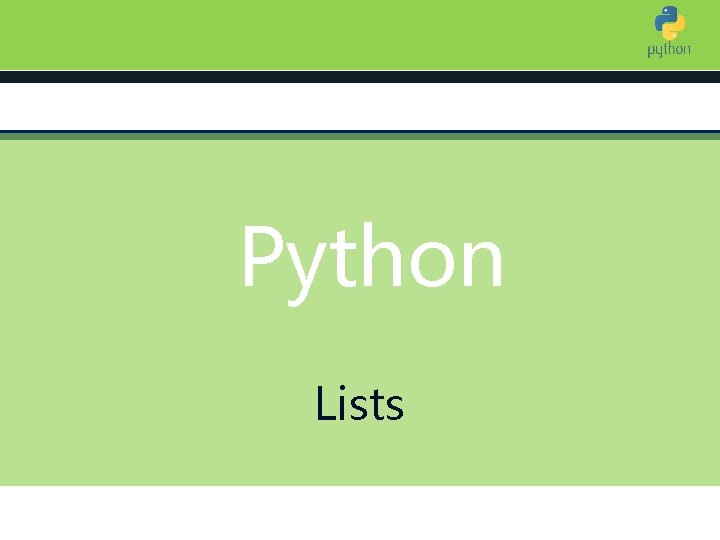Introduction to Python Lists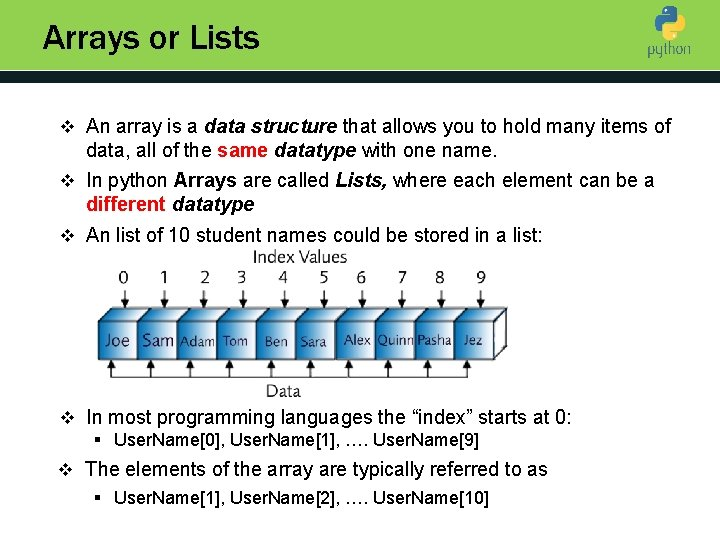Arrays or Lists v An array is a data structure that allows you to hold many items of Introduction to Python data, all of the same datatype with one name. v In python Arrays are called Lists, where each element can be a different datatype v An list of 10 student names could be stored in a list: v In most programming languages the “index” starts at 0: § User. Name, User. Name, …. User. Name v The elements of the array are typically referred to as § User. Name, User. Name, …. User. NameAdvantages of using Lists v Imagine that you want to input, sort and print 100 user names. It Introduction to Python would be very inconvenient to use 100 different variable names v With a list you can have as many data items as you need stored using one variable name v Using an array, you can write: FOR number = 1 TO 100 User. Name[number] = USERINPUT(“ Enter a user name”) NEXT number (Sort the names) FOR number = 1 TO 100 Print (User. Name[number]) Next number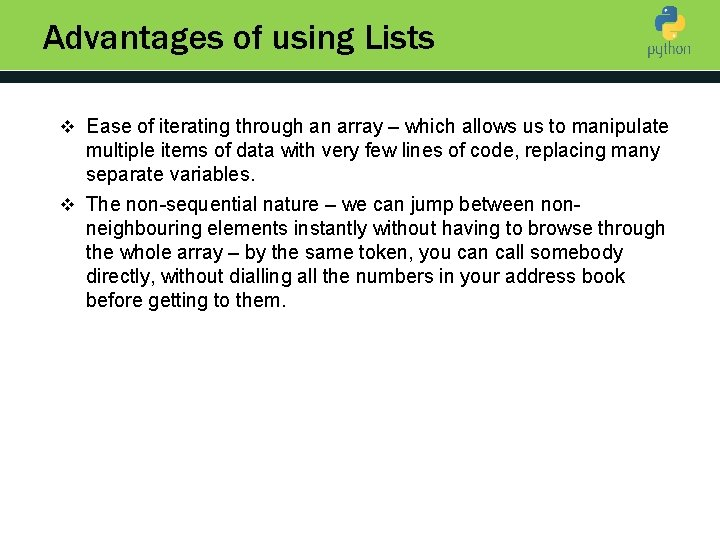Advantages of using Lists v Ease of iterating through an array – which allows us to manipulate Introduction to Python multiple items of data with very few lines of code, replacing many separate variables. v The non-sequential nature – we can jump between non- neighbouring elements instantly without having to browse through the whole array – by the same token, you can call somebody directly, without dialling all the numbers in your address book before getting to them.Recognising Lists or Arrays v Not all languages use square brackets as Python does for indices Introduction to Python v Some use round brackets which might cause confusion because functions and procedures also have round brackets next to their identifiers. v For example in Visual Basic get. Pupil. Data(4) is a function call and Pupils(4) is the 5 th element (assuming zero-based array) of an array Pupils. v In Python you can identify arrays by the index that appears next to array identifiers. E. g. PupilsActivity 1 : spot an array 1. Counter = 3 Introduction to Python 2. Counter = get. Data(5) 3. OUTPUT Widgets 4. Names = find. Max(2) 5. OUTPUT Sentence. SPLIT(“ “) 6. FOR Counter = 1 TO LEN(Lines)-1Activity 1 : spot an array answer 1. Counter = 3 Introduction to Python Just a variable 2. Counter = get. Data(5) A var a function 3. OUTPUT Widgets Widgets is Array! 4. Names = find. Max(2) Names is Array! 5. OUTPUT Sentence. SPLIT(“ “) Array is output 6. FOR Counter = 1 TO LEN(Lines)-1 Lines is an array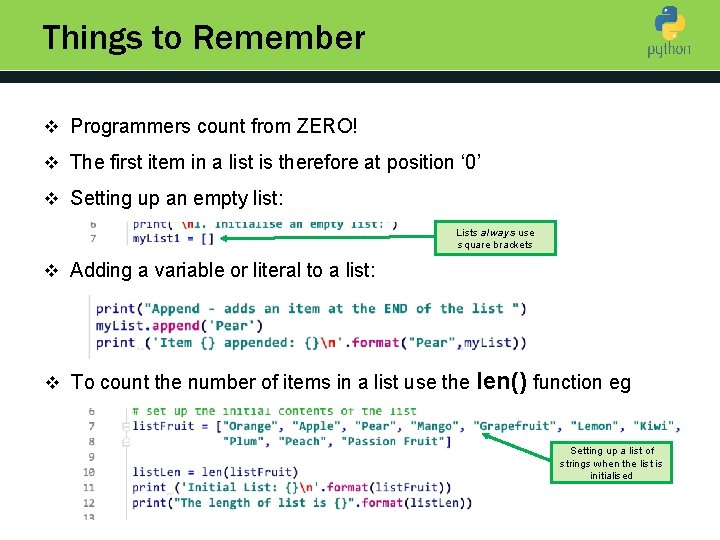Things to Remember v Programmers count from ZERO! Introduction to Python v The first item in a list is therefore at position ‘ 0’ v Setting up an empty list: Lists always use square brackets v Adding a variable or literal to a list: v To count the number of items in a list use the len() function eg Setting up a list of strings when the list is initialised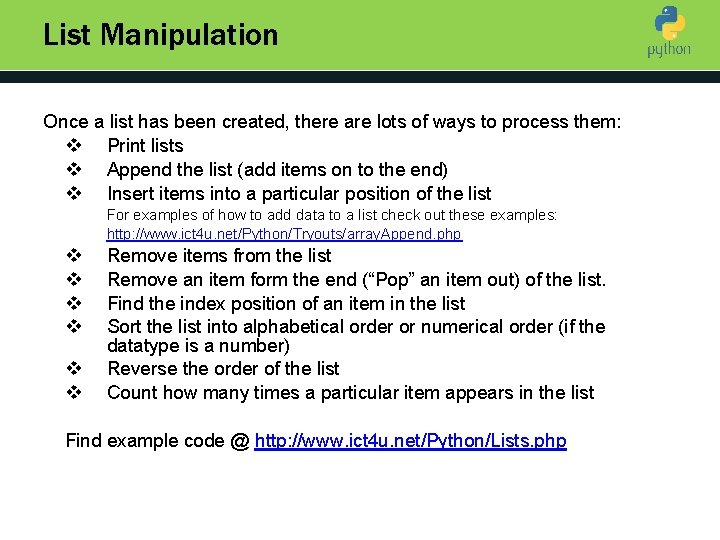List Manipulation Once a list has been created, there are lots of ways to process them: v Print lists Introduction to Python v Append the list (add items on to the end) v Insert items into a particular position of the list For examples of how to add data to a list check out these examples: http: //www. ict 4 u. net/Python/Tryouts/array. Append. php v v v Remove items from the list Remove an item form the end (“Pop” an item out) of the list. Find the index position of an item in the list Sort the list into alphabetical order or numerical order (if the datatype is a number) Reverse the order of the list Count how many times a particular item appears in the list Find example code @ http: //www. ict 4 u. net/Python/Lists. phpActivity 2 Write a program that allows the user to input 5 numbers Introduction to Python that are stored in a list The program outputs the first and the last number. Here is the pseudocode: ARRAY Numbers FOR count = 0 TO 4 OUTPUT ”Data Item” + count INPUT Numbers[count] NEXT count OUTPUT Numbers OUTPUT NumbersActivity 2 - Continued Things to consider: save as For Loop Data Fixed Size Introduction to Python – Initialising the list – Using prompts and input – Datatype of stored data Use this link to open a Python starter code file Here is an example of what the program should look like it when it runs: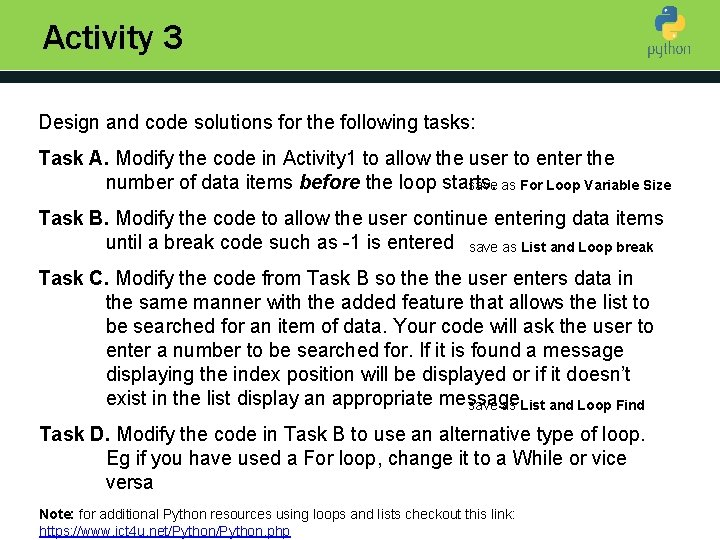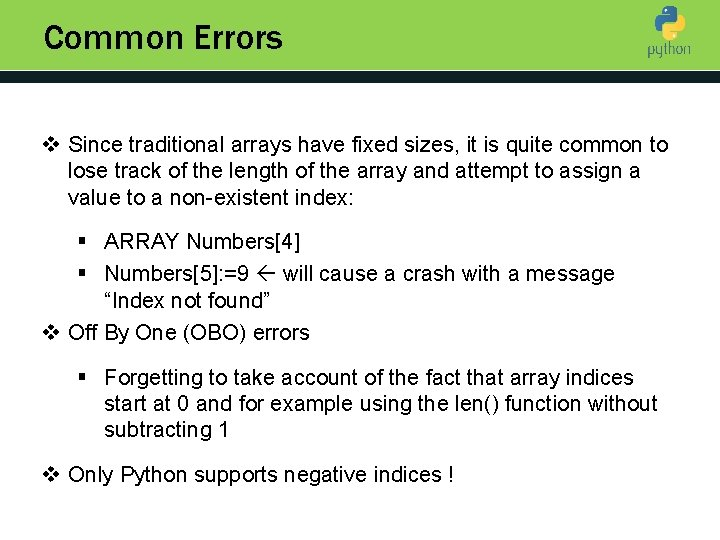Common Errors v Since traditional arrays have fixed sizes, it is quite common to Introduction to Python lose track of the length of the array and attempt to assign a value to a non-existent index: § ARRAY Numbers § Numbers: =9 will cause a crash with a message “Index not found” v Off By One (OBO) errors § Forgetting to take account of the fact that array indices start at 0 and for example using the len() function without subtracting 1 v Only Python supports negative indices !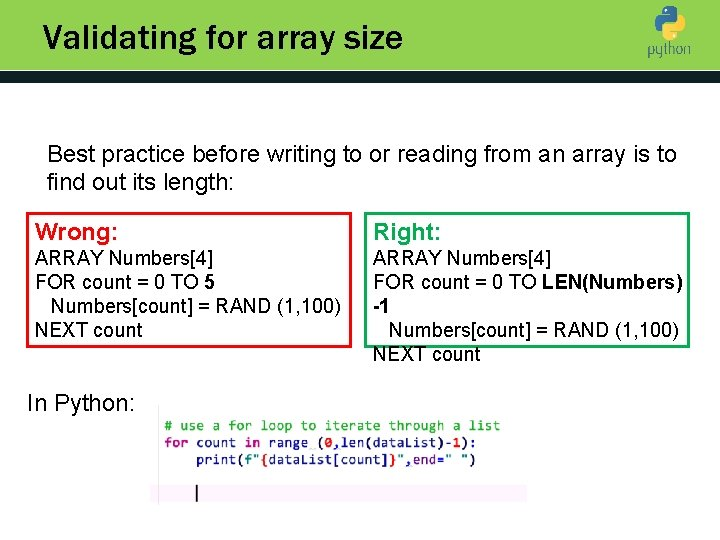Validating for array size Introduction to Python Best practice before writing to or reading from an array is to find out its length: Wrong: Right: ARRAY Numbers FOR count = 0 TO 5 Numbers[count] = RAND (1, 100) NEXT count ARRAY Numbers FOR count = 0 TO LEN(Numbers) -1 Numbers[count] = RAND (1, 100) NEXT count In Python: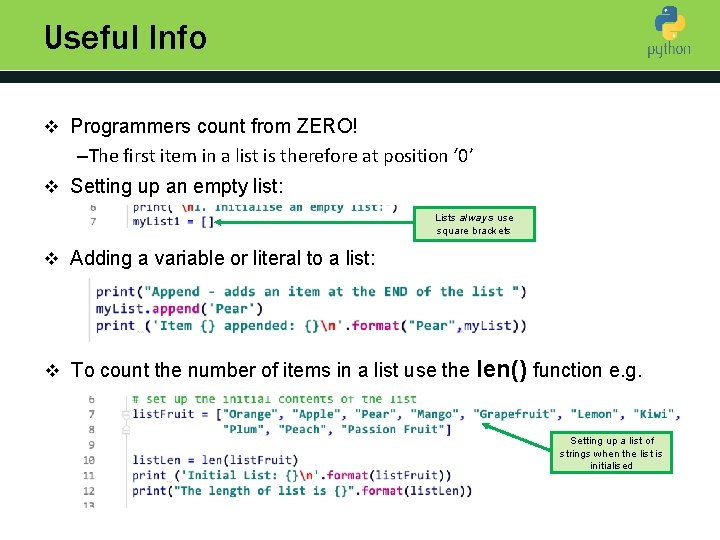Useful Info v Programmers count from ZERO! Introduction to Python –The first item in a list is therefore at position ‘ 0’ v Setting up an empty list: Lists always use square brackets v Adding a variable or literal to a list: v To count the number of items in a list use the len() function e. g. Setting up a list of strings when the list is initialised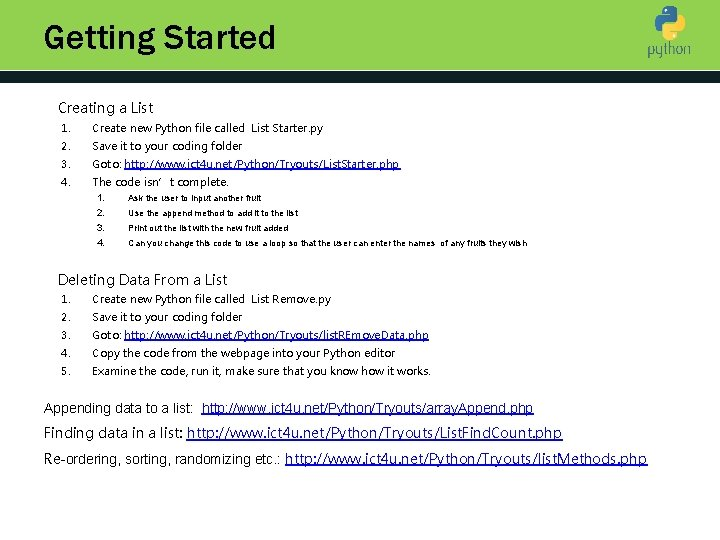Getting Started Creating a List 1. Create new Python file called List Starter. py 2. Save it to your coding folder 3. Goto: http: //www. ict 4 u. net/Python/Tryouts/List. Starter. php 4. The code isn’t complete. Introduction to Python 1. Ask the user to input another fruit 2. Use the append method to add it to the list 3. Print out the list with the new fruit added 4. Can you change this code to use a loop so that the user can enter the names of any fruits they wish Deleting Data From a List 1. Create new Python file called List Remove. py 2. Save it to your coding folder 3. Goto: http: //www. ict 4 u. net/Python/Tryouts/list. REmove. Data. php 4. Copy the code from the webpage into your Python editor 5. Examine the code, run it, make sure that you know how it works. Appending data to a list: http: //www. ict 4 u. net/Python/Tryouts/array. Append. php Finding data in a list: http: //www. ict 4 u. net/Python/Tryouts/List. Find. Count. php Re-ordering, sorting, randomizing etc. : http: //www. ict 4 u. net/Python/Tryouts/list. Methods. php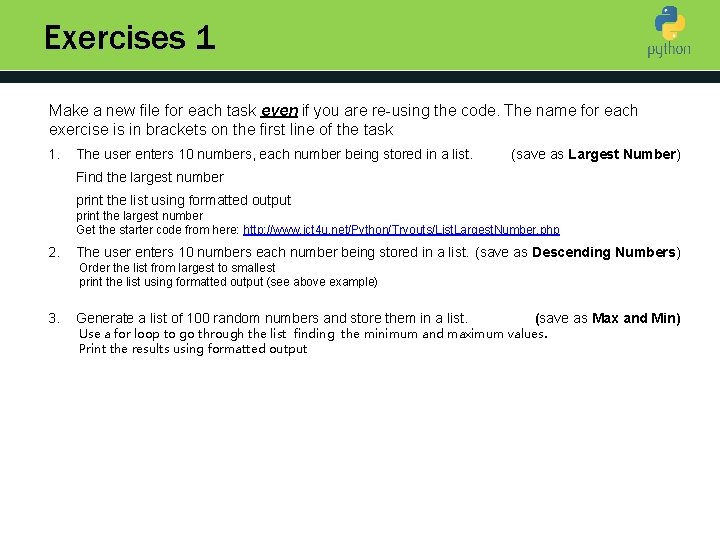Exercises 1 Make a new file for each task even if you are re-using the code. The name for each exercise is in brackets on the first line of the task 1. Introduction to Python (save as Largest Number) The user enters 10 numbers, each number being stored in a list. Find the largest number print the list using formatted output print the largest number Get the starter code from here: http: //www. ict 4 u. net/Python/Tryouts/List. Largest. Number. php 2. The user enters 10 numbers each number being stored in a list. (save as Descending Numbers) Order the list from largest to smallest print the list using formatted output (see above example) 3. Generate a list of 100 random numbers and store them in a list. (save as Max and Min) Use a for loop to go through the list finding the minimum and maximum values. Print the results using formatted outputExercises 2 1. The user enters 10 names each being stored in a list. 2. Using the names list from the previous exercise 3. Using the name list from the previous exercise (save as Position in List) 4. Using the name list from the previous exercise. (save as Remove a Name) 5. Copy the starter code from this link: Strings and Numbers Reverse the order of the list print the list using formatted output (save as Names Reversed) Introduction to Python (save as Find a Name) Prompt the user for a name to find Count how many times the name appears in the list. Display the name and the number of times it was found Prompt the user for a name If the name is in the list display the name and the index position otherwise display the name and a message that it was not in the list print the list using formatted output Remove the 0 and 4 th items. After each item has been removed, print the list using formatted output and the current length of the list Prompt the user for a name and then remove it from the list print the list using formatted output and the current length of the list (save as Strings and Numbers) Use the hints in the code to create separate lists of strings and numbers. Sort the strings list in ascending order – print the results using formatted output Sort the strings list in descending order - print the results using formatted output Choose one item at random from the list of strings and print it out Sort the number list from lowest to highest - print the results using formatted output Sort the number list from highest to lowest - print the results using formatted output Randomise the list of numbers. print the results using formatted outputExercises 3 1. Adam wants to record the goals scored by his favourite football team. 2. Adam wants to know how well his team is doing. 3. Things are going well. Adam wants to compare this season with last Compare) (save as Football Team) Create a python program that starts with an empty list and allows him to enter as many scores until -1 is entered Introduction to Python Print all the entries recorded in a formatted manner (save as Football Analysis) Modify the Football Team program to count all the numbers in the list, sum their values and work out the average. After all the scores have been printed also show the number of times goals were scored (count), the total goals (sum) and average print the list using formatted manner and the current length of the list (save as Football Last season Adam’s recorded the following goal scores for his team: 4, 2, 5, 1, 5, 3, 2, 7 Modify your program so that it starts with last season’s scores already stored in one list When this seasons scores have been entered merge the two lists and sort them into ascending order. Print the results in a formatted manner as for the previous task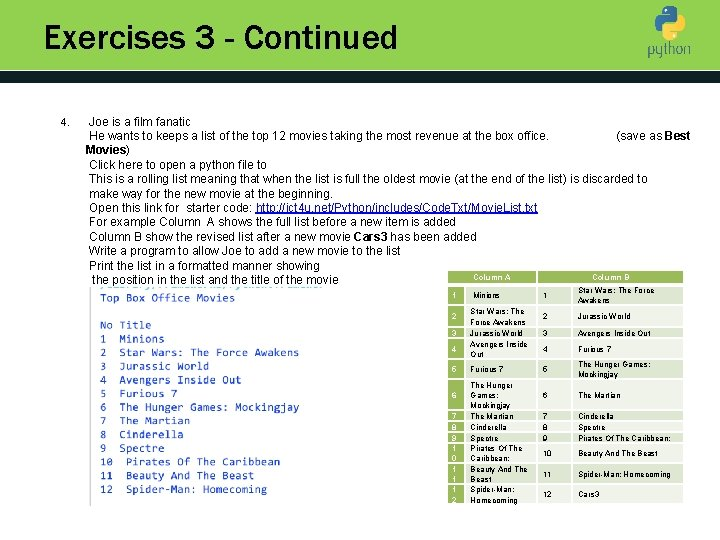Exercises 3 - Continued 4. Joe is a film fanatic He wants to keeps a list of the top 12 movies taking the most revenue at the box office. (save as Best Introduction to Python Movies) Click here to open a python file to This is a rolling list meaning that when the list is full the oldest movie (at the end of the list) is discarded to make way for the new movie at the beginning. Open this link for starter code: http: //ict 4 u. net/Python/includes/Code. Txt/Movie. List. txt For example Column A shows the full list before a new item is added Column B show the revised list after a new movie Cars 3 has been added Write a program to allow Joe to add a new movie to the list Print the list in a formatted manner showing Column A Column B the position in the list and the title of the movie 1 2 3 4 5 6 7 8 9 1 0 1 1 1 2 Minions Star Wars: The Force Awakens Jurassic World Avengers Inside Out Furious 7 The Hunger Games: Mockingjay The Martian Cinderella Spectre Pirates Of The Caribbean: Beauty And The Beast Spider-Man: Homecoming 1 Star Wars: The Force Awakens 2 Jurassic World 3 Avengers Inside Out 4 Furious 7 5 The Hunger Games: Mockingjay 6 The Martian 7 8 9 Cinderella Spectre Pirates Of The Caribbean: 10 Beauty And The Beast 11 Spider-Man: Homecoming 12 Cars 3Printing a List in Python Introduction to Python The whole list is printed without any formatting Use a for loop to iterate through the list Then each item can be formatted in the output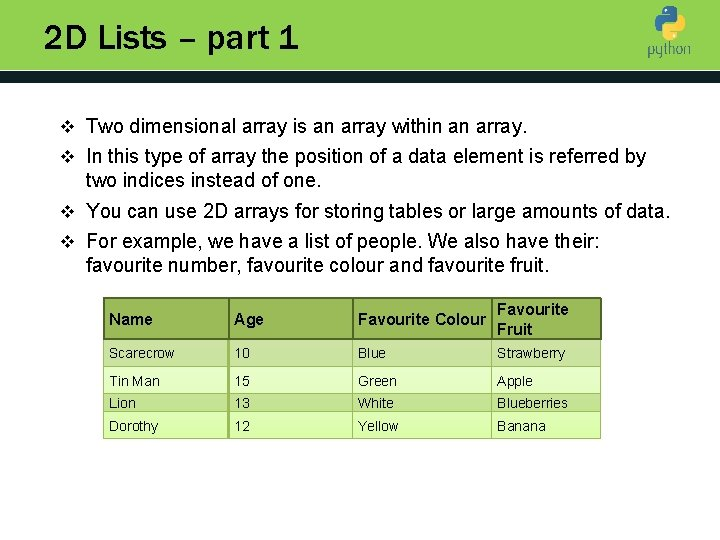2 D Lists – part 1 v Two dimensional array is an array within an array. Introduction to Python v In this type of array the position of a data element is referred by two indices instead of one. v You can use 2 D arrays for storing tables or large amounts of data. v For example, we have a list of people. We also have their: favourite number, favourite colour and favourite fruit. Name Age Favourite Colour Favourite Fruit Scarecrow 10 Blue Strawberry Tin Man 15 Green Apple Lion 13 White Blueberries Dorothy 12 Yellow Banana2 D Lists – part 2 There are three ways of storing this: 1. Introduction to Python Using a different variables for piece of data: first. Name = ‘Scarecrow’ first. Fav. Number = 10 first. Colour = ‘Blue’ first. Fruit = ‘Strawberry’ second. Name = ‘Tin Man’ second. Fav. Number = 15 etc This is not satisfactory as it takes a long time to type out each variable, is difficult to process and can be confusing at times. 2. Using Single List for each person e. g. in the order of name, favourite number, favourite colour, favourite fruit first = [‘Scarecrow’, 10, ‘Blue’, ‘Strawberry’] second = [‘Tin Man’, 15, ‘Green’, ‘Apple’] etc This is also not a preferred method. The data can get confusing, and it is difficult to add data for another person. It is still difficult to process.2 D Lists – part 3 2. Merge all of single array lists into one big 2 D array list. In the order of name, favourite number, favourite colour, favourite fruit: Introduction to Python list. Favorites = [ [Scarecrow', 10, 'Blue', 'Strawberry'], [Tin Man', 15, 'Green', 'Apple'], [Lion', 13, 'White', 'Blueberries'], ['Dorothy', 12, 'Yellow', 'Banana'] ] First dwarf Element  4 th piece of data for this element  v To pick a value from the 2 d array, reference it with two square brackets. v In the first, you enter the ‘record’, in the second you enter the ‘field’ of the larger list. v For example, the favourite colour of the second entry: print(list. Favourites) will return Green, Tin Man’s favourite colour.Exercise 1 - Airports are allocated a three letter code. Save as Airport Codes The airlines system stores the airport name and corresponding airport codes. Introduction to Python You have to write a program that accepts an airport code and displays the corresponding airport name, using the data in the table below. If an airport code that is not in the table is entered an appropriate error message should be given To complete this exercise, you should write a modular program that has at least two functions Use this starter code to begin your program Code Name BCN Barcelona DUB Dublin LAX Los Angeles CDG Paris, Charles De Gaulle PRG Prague LHR London Heathrow FCO Rome, Fiumicino GOT Gothenburg, Sweden JFK New York, John F Kennedy WAS Washington Dulles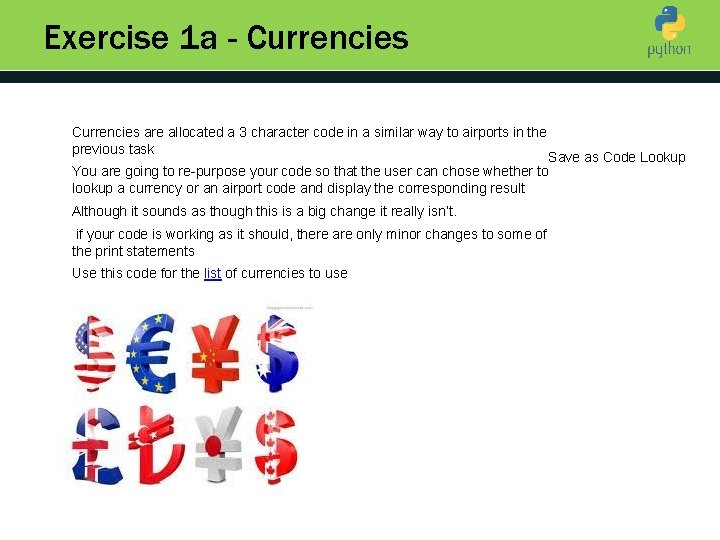Exercise 1 a - Currencies are allocated a 3 character code in a similar way to airports in the Introduction to Python previous task Save as Code Lookup You are going to re-purpose your code so that the user can chose whether to lookup a currency or an airport code and display the corresponding result Although it sounds as though this is a big change it really isn’t. if your code is working as it should, there are only minor changes to some of the print statements Use this code for the list of currencies to use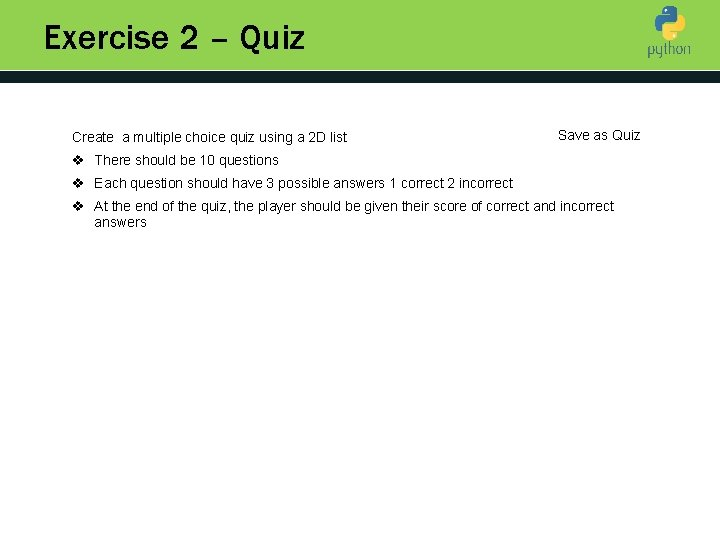Exercise 2 – Quiz Create a multiple choice quiz using a 2 D list v There should be 10 questions Introduction to Python Save as Quiz v Each question should have 3 possible answers 1 correct 2 incorrect v At the end of the quiz, the player should be given their score of correct and incorrect answers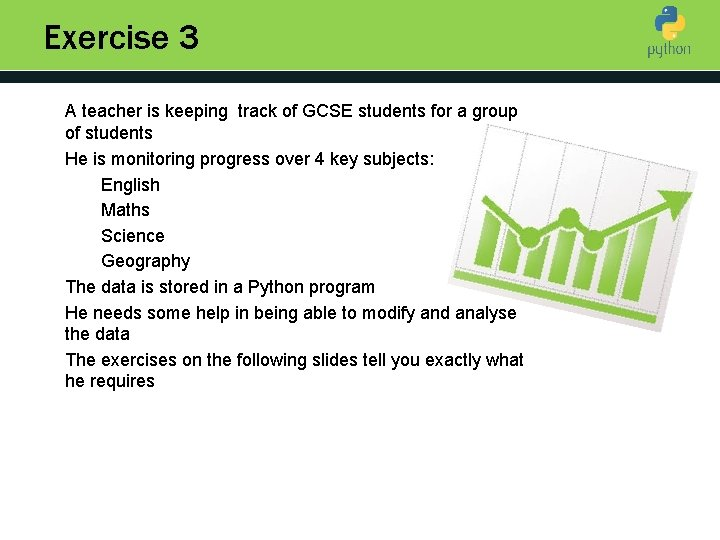Exercise 3 A teacher is keeping track of GCSE students for a group of students Introduction to Python He is monitoring progress over 4 key subjects: English Maths Science Geography The data is stored in a Python program He needs some help in being able to modify and analyse the data The exercises on the following slides tell you exactly what he requires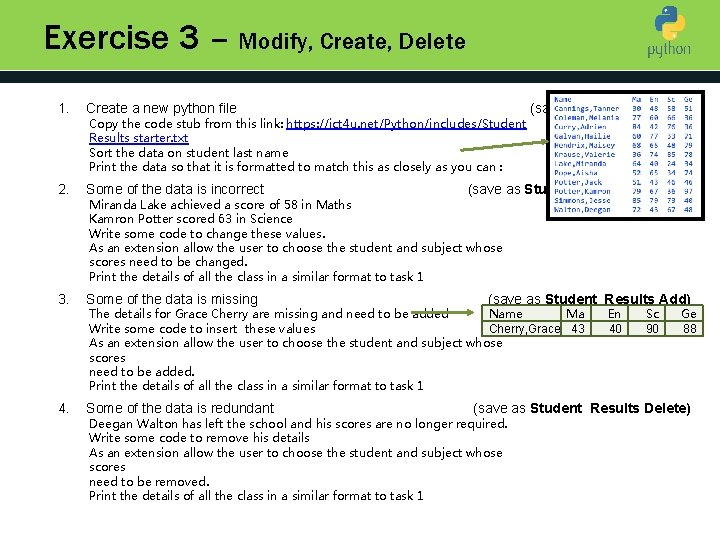Exercise 3 – Modify, Create, Delete 1. Create a new python file 2. Some of the data is incorrect 3. Some of the data is missing 4. Some of the data is redundant Copy the code stub from this link: https: //ict 4 u. net/Python/includes/Student Results starter. txt Sort the data on student last name Introduction to Python Print the data so that it is formatted to match this as closely as you can : (save as Student Results) (save as Student Results Update) Miranda Lake achieved a score of 58 in Maths Kamron Potter scored 63 in Science Write some code to change these values. As an extension allow the user to choose the student and subject whose scores need to be changed. Print the details of all the class in a similar format to task 1 (save as Student Results Add) Name Ma The details for Grace Cherry are missing and need to be added Write some code to insert these values Cherry, Grace 43 As an extension allow the user to choose the student and subject whose scores need to be added. Print the details of all the class in a similar format to task 1 En 40 Sc 90 Ge 88 (save as Student Results Delete) Deegan Walton has left the school and his scores are no longer required. Write some code to remove his details As an extension allow the user to choose the student and subject whose scores need to be removed. Print the details of all the class in a similar format to task 1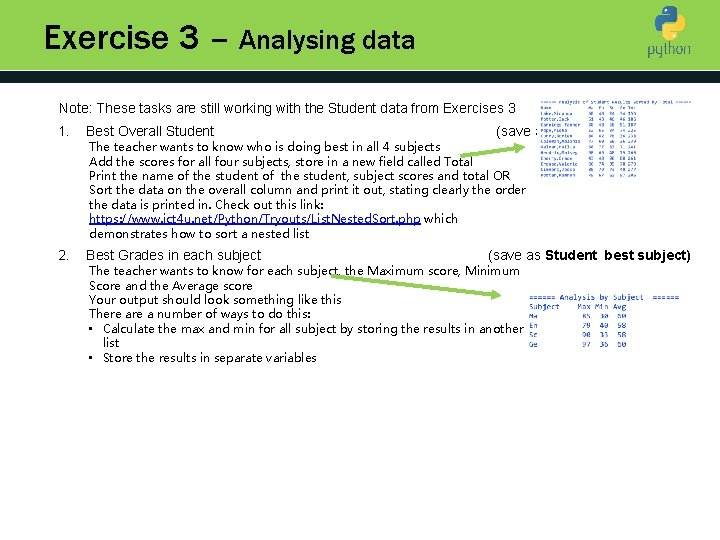Exercise 3 – Analysing data Note: These tasks are still working with the Student data from Exercises 3 1. Best Overall Student 2. Best Grades in each subject (save as Student Best Overall ) The teacher wants to know who is doing best in all 4 subjects Introduction to Python Add the scores for all four subjects, store in a new field called Total Print the name of the student, subject scores and total OR Sort the data on the overall column and print it out, stating clearly the order the data is printed in. Check out this link: https: //www. ict 4 u. net/Python/Tryouts/List. Nested. Sort. php which demonstrates how to sort a nested list (save as Student best subject) The teacher wants to know for each subject, the Maximum score, Minimum Score and the Average score Your output should look something like this There a number of ways to do this: • Calculate the max and min for all subject by storing the results in another list • Store the results in separate variablesBig picture Introduction to Python v Arrays are made up of elements (items) holding related data. v Every item in an array has an index – like a room number in a hotel that we can access it by. v Some arrays can have “multiple floors” – then we call them “two-dimensional” or “nested”. To write or read an item, we provide its floor (row) first and its room number on that floor (column).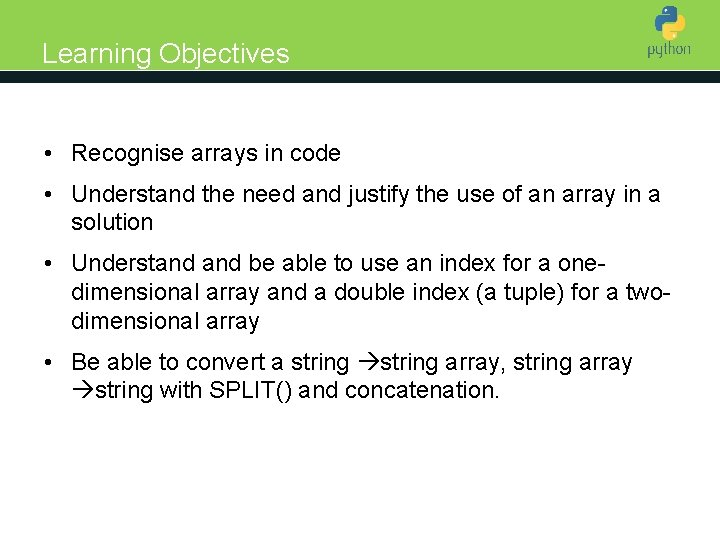Learning Objectives Introduction to Python • Recognise arrays in code • Understand the need and justify the use of an array in a solution • Understand be able to use an index for a onedimensional array and a double index (a tuple) for a twodimensional array • Be able to convert a string array, string array string with SPLIT() and concatenation.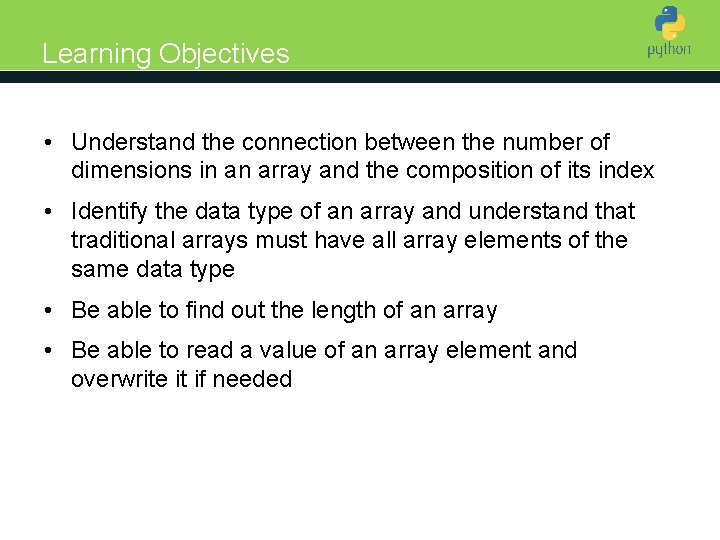Learning Objectives • Understand the connection between the number of Introduction to Python dimensions in an array and the composition of its index • Identify the data type of an array and understand that traditional arrays must have all array elements of the same data type • Be able to find out the length of an array • Be able to read a value of an array element and overwrite it if needed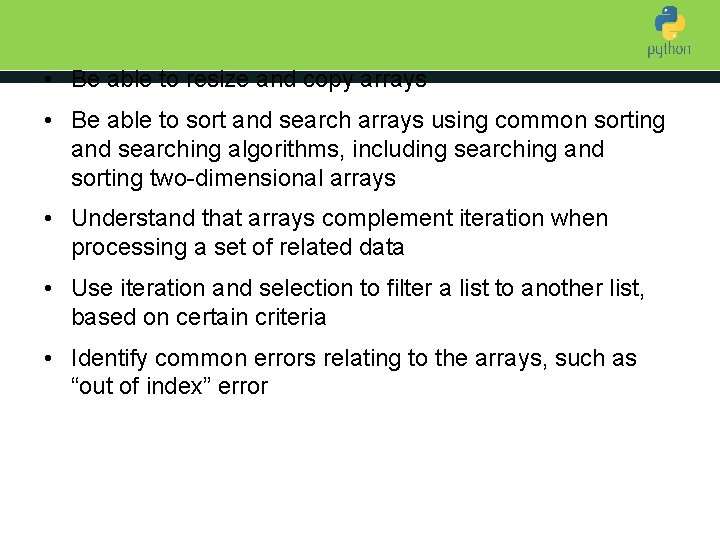• Be able to resize and copy arrays • Be able to sort and search arrays using common sorting Introduction to Python and searching algorithms, including searching and sorting two-dimensional arrays • Understand that arrays complement iteration when processing a set of related data • Use iteration and selection to filter a list to another list, based on certain criteria • Identify common errors relating to the arrays, such as “out of index” errorWhat are arrays? Introduction to Python • An array is an ordered collection of related data where each element is accessible by a number, known as an index. • Arrays usually have fixed sizes and occupy a fixed (static) amount of memory. N-1 is the last index INDE X 0 VALU 45 E 1 2 87 23 3 4 5 6 7 101 2 42 11 92 Array item/elementWhat are they good for? Introduction to Python • Ease of iterating through an array – which allows us to manipulate multiple items of data with very few lines of code, replacing many separate variables. • The non-sequential nature – we can jump between nonneighbouring elements instantly without having to browse through the whole array – by the same token, you can call somebody directly, without dialling all the numbers in your book before getting to them.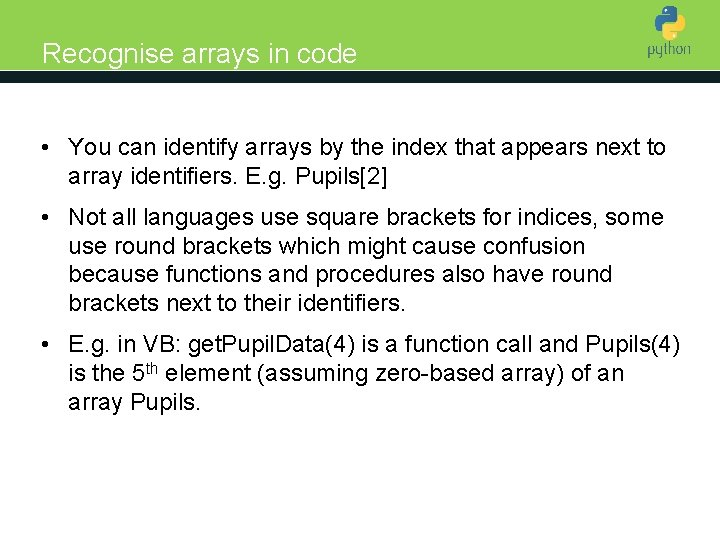Recognise arrays in code Introduction to Python • You can identify arrays by the index that appears next to array identifiers. E. g. Pupils • Not all languages use square brackets for indices, some use round brackets which might cause confusion because functions and procedures also have round brackets next to their identifiers. • E. g. in VB: get. Pupil. Data(4) is a function call and Pupils(4) is the 5 th element (assuming zero-based array) of an array Pupils.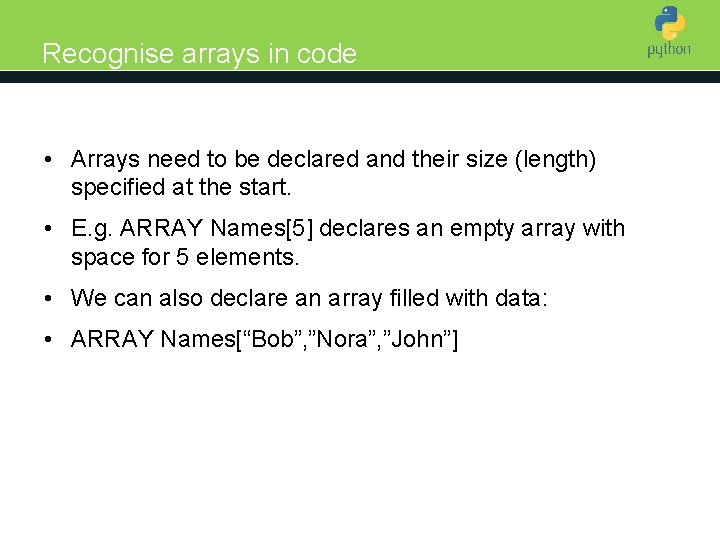Recognise arrays in code Introduction to Python • Arrays need to be declared and their size (length) specified at the start. • E. g. ARRAY Names declares an empty array with space for 5 elements. • We can also declare an array filled with data: • ARRAY Names[“Bob”, ”Nora”, ”John”]Other ways to identify Introduction to Python • It also helps that array identifiers (names) themselves, are in plural, e. g. Pupils not Pupil. • We should also expect array indices to be integers, so any other data type in brackets is not an array. • Some arrays are automatically created, for example by a string SPLIT function which creates an array of string data.Activity 1 : spot an array 1. Counter: =3 Introduction to Python 2. Counter: =get. Data(5) 3. OUTPUT Widgets 4. Names: =find. Max(2) 5. OUTPUT Sentence. SPLIT(“ “) 6. FOR Counter=1 TO LEN(Lines)-1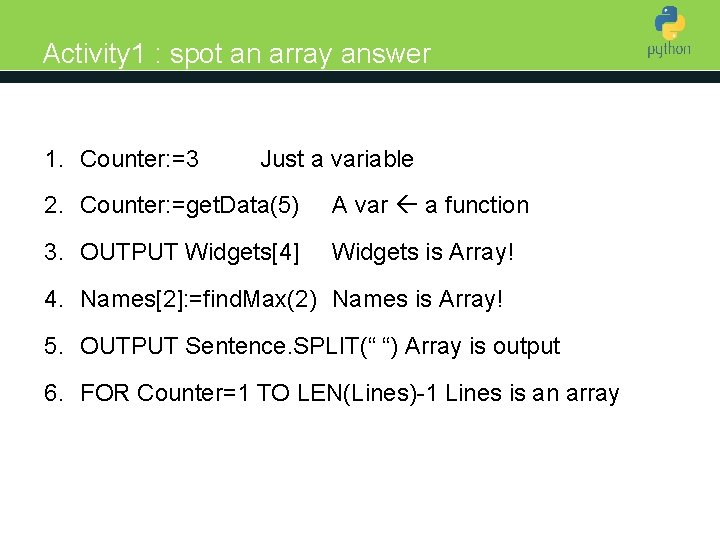Activity 1 : spot an array answer 1. Counter: =3 Introduction to Python Just a variable 2. Counter: =get. Data(5) A var a function 3. OUTPUT Widgets Widgets is Array! 4. Names: =find. Max(2) Names is Array! 5. OUTPUT Sentence. SPLIT(“ “) Array is output 6. FOR Counter=1 TO LEN(Lines)-1 Lines is an arrayZero or one-based? Introduction to Python • We should also note that in most programming languages, lists/arrays begin with zero, in other words, the first element is said to have an “index of zero”. This is similar to how we count building floors in this country, the first floor isn’t actually the first, it’s on top of the ground floor, which can be said to have an index of zero.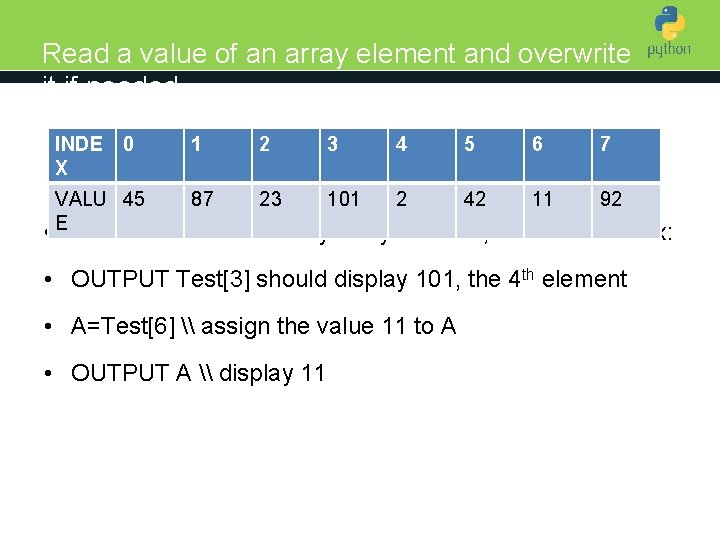Read a value of an array element and overwrite it if needed INDE X 0 1 2 3 4 to Python 5 Introduction 6 7 VALU 45 87 23 101 2 42 11 92 • E To read the value of any array element, we use its index: • OUTPUT Test should display 101, the 4 th element • A=Test \ assign the value 11 to A • OUTPUT A \ display 11Find out the length of an array Introduction to Python • All languages support keywords to find the length of an array. • We commonly code it like so: • ARRAY Items • how. Many: =LEN(Items) • It is commonly used in FOR loops while iterating through array elements.Use of an array in a solution Introduction to Python • We will design a solution that takes 4 numbers from a user and outputs the first and the last number • Extension: this time it outputs the sum of the second and third number.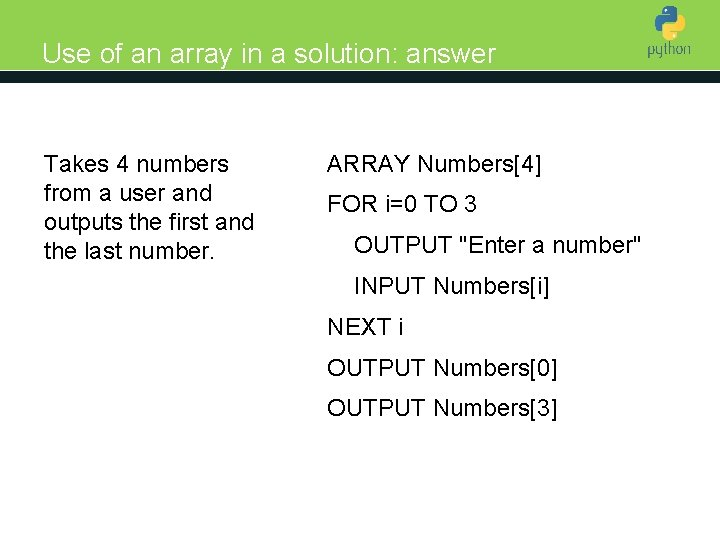Use of an array in a solution: answer Takes 4 numbers from a user and outputs the first and the last number. Introduction to Python ARRAY Numbers FOR i=0 TO 3 OUTPUT "Enter a number" INPUT Numbers[i] NEXT i OUTPUT Numbers OUTPUT NumbersUse of an array in a solution: answer Outputs the sum of the second and third number. Introduction to Python ARRAY Numbers FOR i=0 TO 3 OUTPUT "Enter a number" INPUT Numbers[i] NEXT i Sum: = Numbers + Numbers OUTPUT SumActivity 2: Introduction to Python • We will design a solution that takes 4 numbers from a user and outputs their sum, max and min. • Extension: repeat this task but only for the 3 largest items out of this array.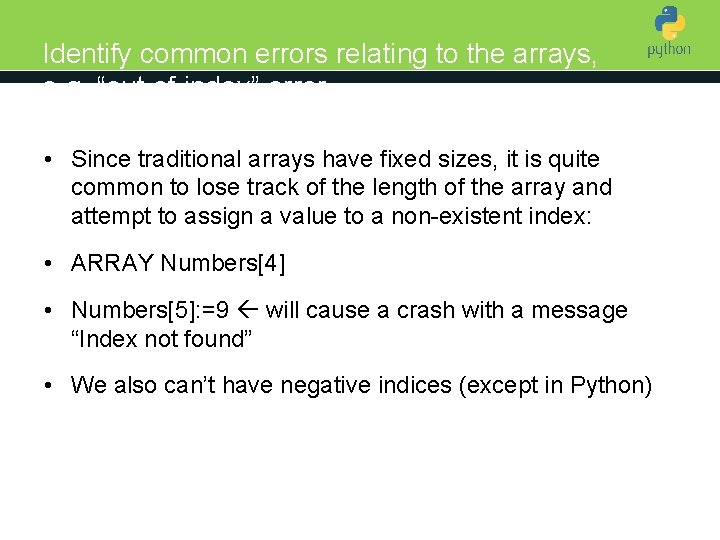Identify common errors relating to the arrays, e. g. “out of index” error Introduction to Python • Since traditional arrays have fixed sizes, it is quite common to lose track of the length of the array and attempt to assign a value to a non-existent index: • ARRAY Numbers • Numbers: =9 will cause a crash with a message “Index not found” • We also can’t have negative indices (except in Python)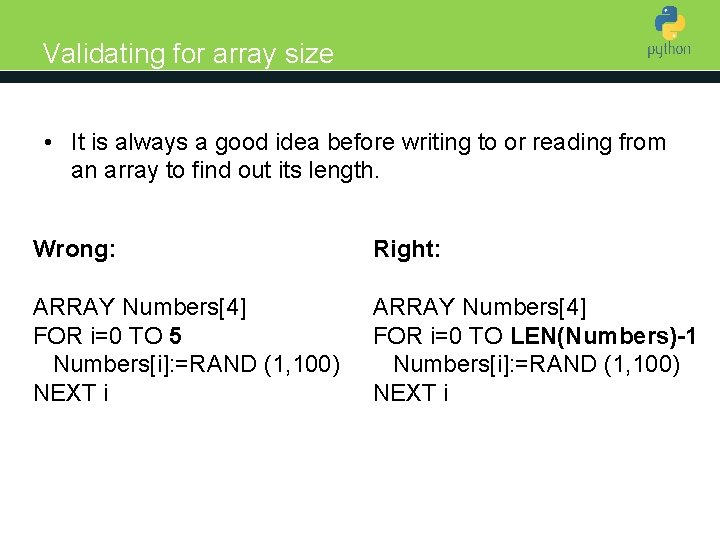Validating for array size • It is always a good idea before writing to or reading from Introduction to Python an array to find out its length. Wrong: Right: ARRAY Numbers FOR i=0 TO 5 Numbers[i]: =RAND (1, 100) NEXT i ARRAY Numbers FOR i=0 TO LEN(Numbers)-1 Numbers[i]: =RAND (1, 100) NEXT i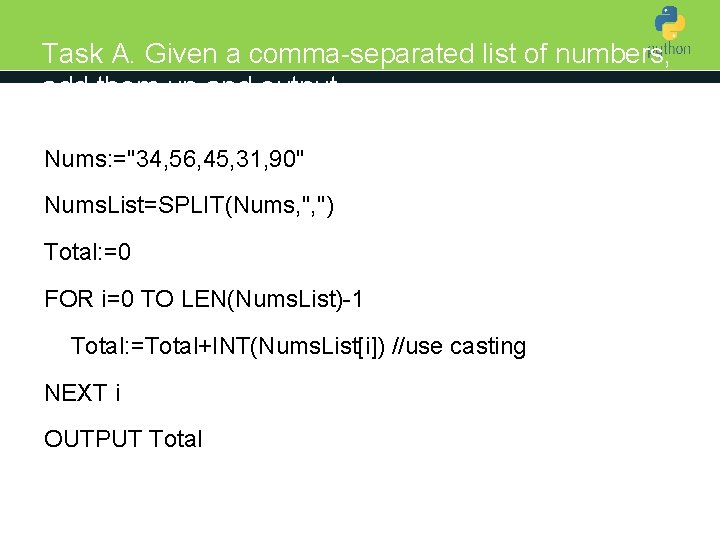Task A. Given a comma-separated list of numbers, add them up and output Introduction to Python Nums: ="34, 56, 45, 31, 90" Nums. List=SPLIT(Nums, ", ") Total: =0 FOR i=0 TO LEN(Nums. List)-1 Total: =Total+INT(Nums. List[i]) //use casting NEXT i OUTPUT Total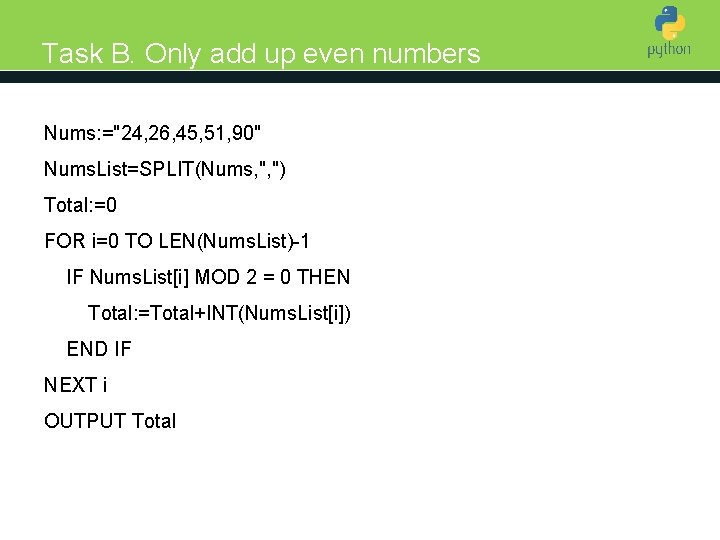Task B. Only add up even numbers Nums: ="24, 26, 45, 51, 90" Introduction to Python Nums. List=SPLIT(Nums, ", ") Total: =0 FOR i=0 TO LEN(Nums. List)-1 IF Nums. List[i] MOD 2 = 0 THEN Total: =Total+INT(Nums. List[i]) END IF NEXT i OUTPUT TotalTask C. Only add up every other number Nums: ="24, 26, 45, 51, 90"Introduction to Python Nums. List=SPLIT(Nums, ", ") Total: =0 FOR i=0 TO LEN(Nums. List)-1 STEP 2 Total: =Total+INT(Nums. List[i]) NEXT i OUTPUT Total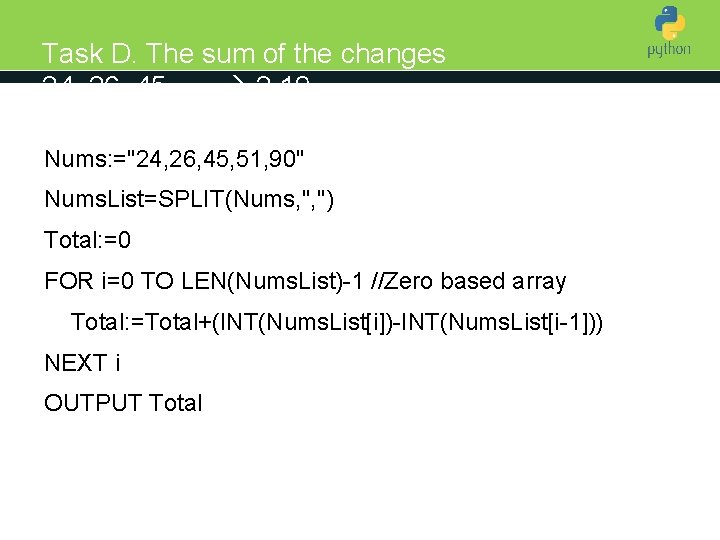Task D. The sum of the changes 24, 26, 45, . . 2, 19, . . . Introduction to Python Nums: ="24, 26, 45, 51, 90" Nums. List=SPLIT(Nums, ", ") Total: =0 FOR i=0 TO LEN(Nums. List)-1 //Zero based array Total: =Total+(INT(Nums. List[i])-INT(Nums. List[i-1])) NEXT i OUTPUT Total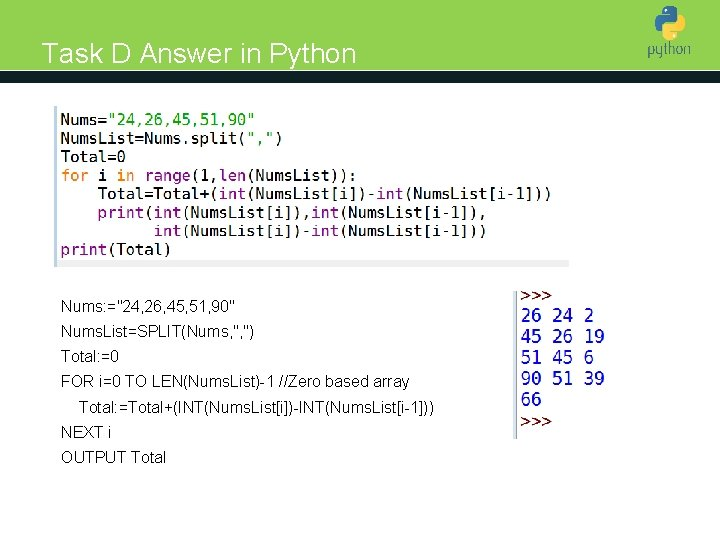Task D Answer in Python Introduction to Python Nums: ="24, 26, 45, 51, 90" Nums. List=SPLIT(Nums, ", ") Total: =0 FOR i=0 TO LEN(Nums. List)-1 //Zero based array Total: =Total+(INT(Nums. List[i])-INT(Nums. List[i-1])) NEXT i OUTPUT Total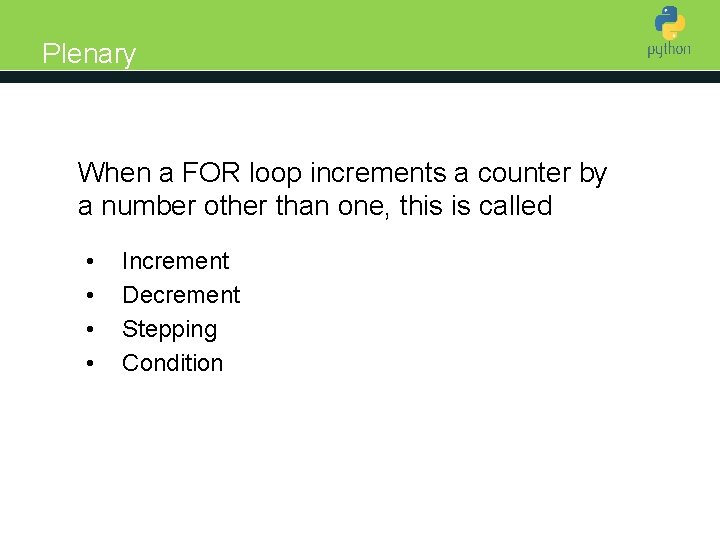Plenary Introduction to Python When a FOR loop increments a counter by a number other than one, this is called • • Increment Decrement Stepping ConditionPlenary Introduction to Python Which of the following is not true about arrays : • All array elements have the same data type • Arrays have fixed size that can’t be easily changed during a program run • Names[LEN(Names)] is the last element of the array Names • The use of iteration makes arrays less relevant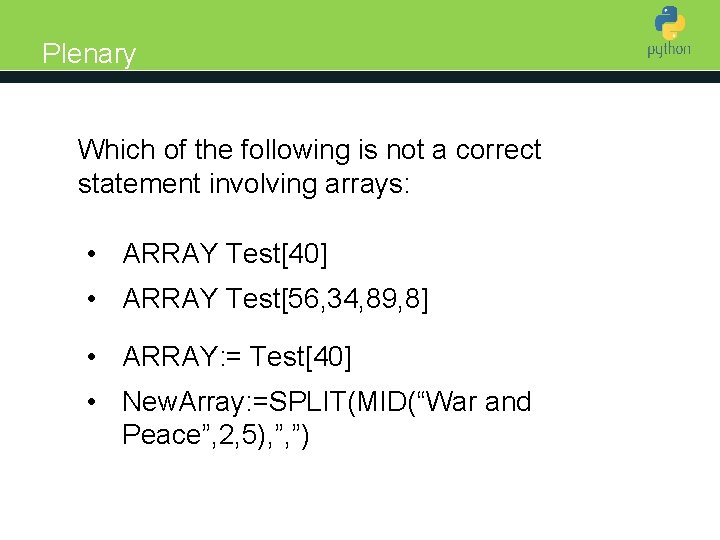Plenary Introduction to Python Which of the following is not a correct statement involving arrays: • ARRAY Test • ARRAY Test[56, 34, 89, 8] • ARRAY: = Test • New. Array: =SPLIT(MID(“War and Peace”, 2, 5), ”, ”)Plenary Introduction to Python Which of the following is not a correct statement involving arrays: • J: =Pupils[5, 8] • Nums[i, j]: =Nums[i-1, j] • Nums[i, j]: =Nums[i-1] • B: =get. Choices(45)